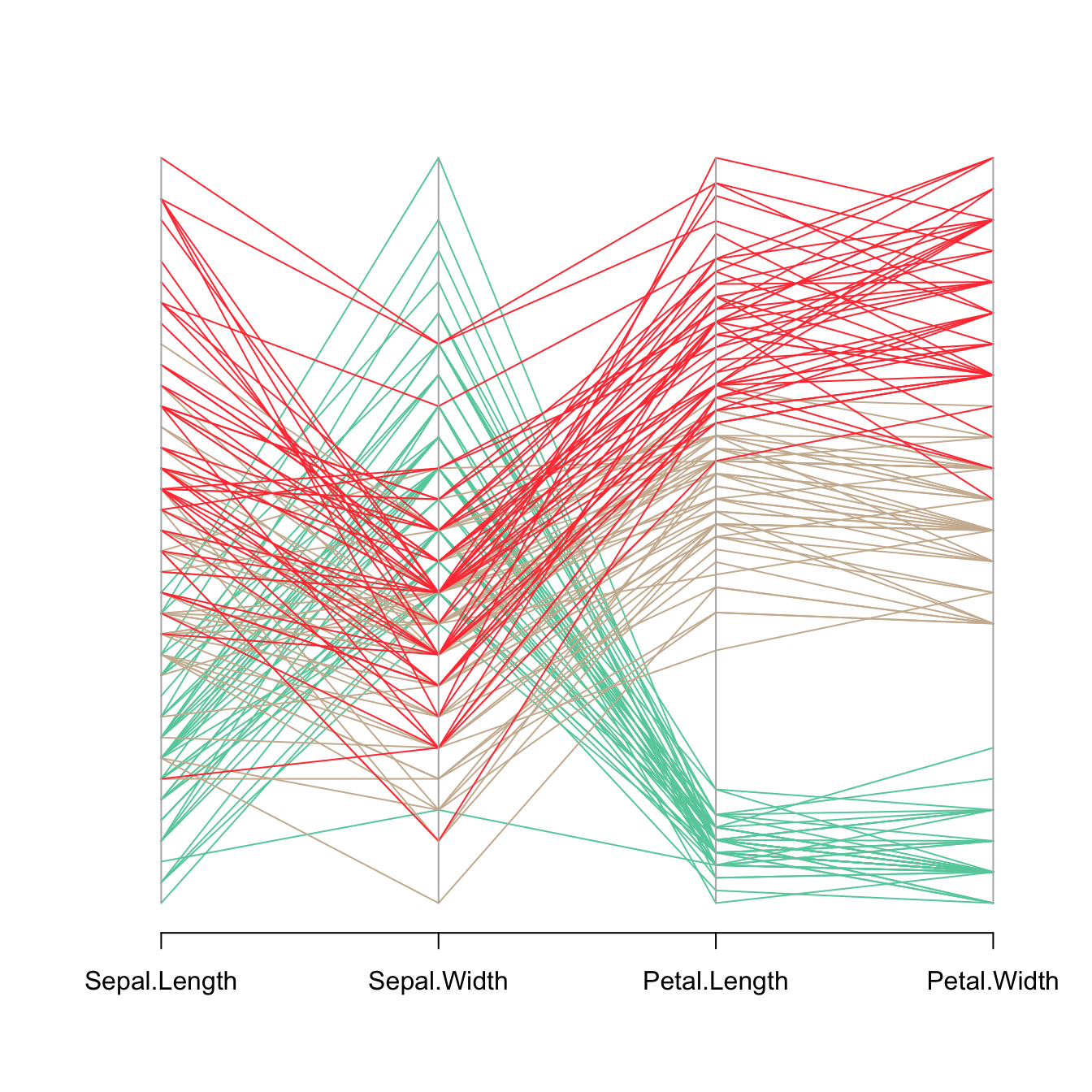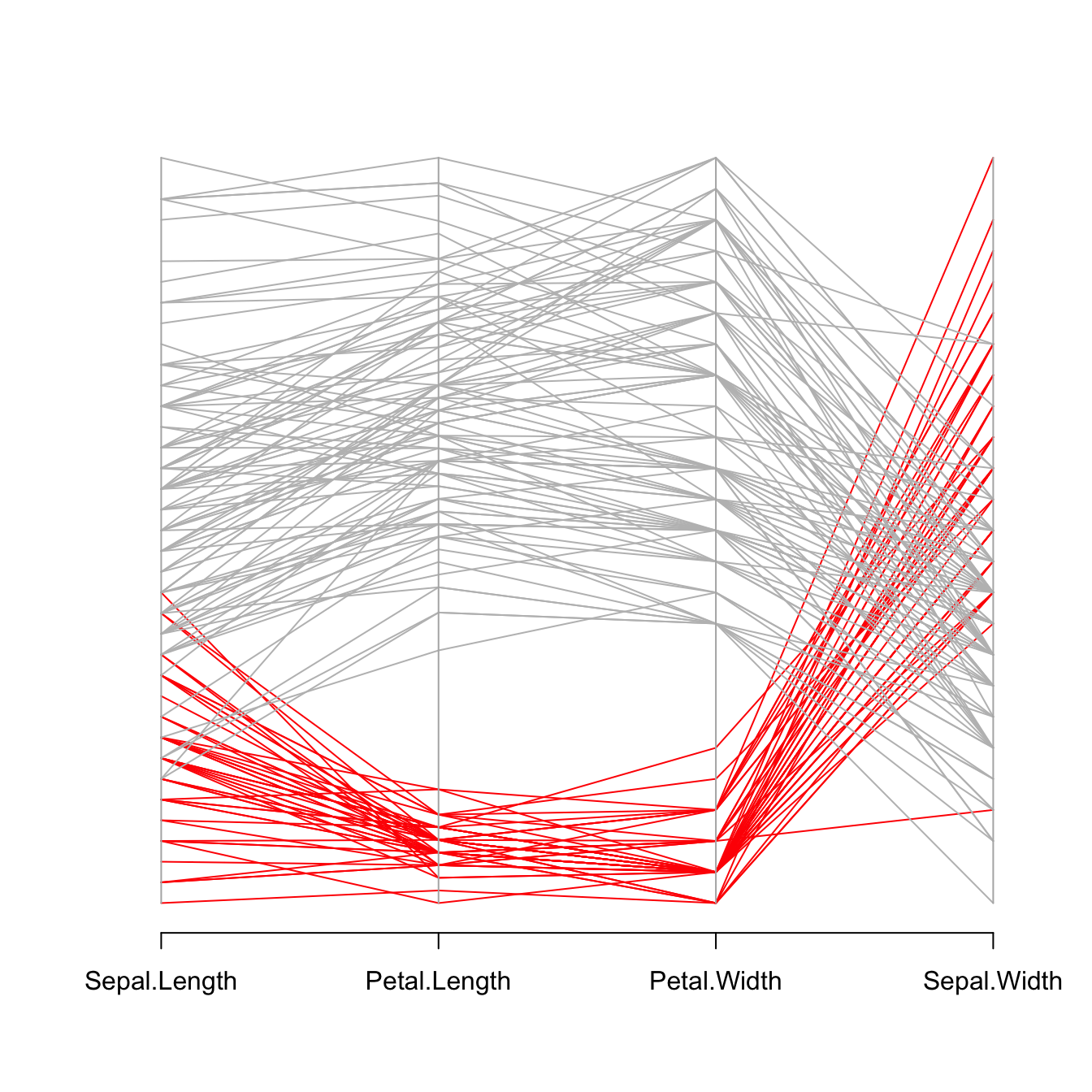# Parallel chart with the MASS library

This post explains how to build a parallel coordinate chart with `R` and the `MASS` library. Note that using ggplot2 is probably a better option.

# The `parcoord()` function of the `MASS` library.

The `MASS` library provides the `parcoord()` function that automatically builds parallel coordinates chart.

The input dataset must be a data frame composed by numeric variables only. Each variable will be used to build one vertical axis of the chart.``````# You need the MASS library
library(MASS)

# Vector color
my_colors <- colors()[as.numeric(iris\$Species)*11]

# Make the graph !
parcoord(iris[,c(1:4)] , col= my_colors  )``````

# Reorder variables

It is important to find the best variable order in your parallel coordinates chart. To change it, just change the order in the input dataset.

Note: the `RColorBrewer` package is used to generate a nice and reliable color palette.``````# You need the MASS library
library(MASS)

# Vector color
library(RColorBrewer)
palette <- brewer.pal(3, "Set1")
my_colors <- palette[as.numeric(iris\$Species)]

# Make the graph !
parcoord(iris[,c(1,3,4,2)] , col= my_colors  )``````

# Highlight a group

Data visualization aims to highlight a story in the data. If you are interested in a specific group, you can highlight it as follow:``````# You need the MASS library
library(MASS)

# Let's use the Iris dataset as an example
data(iris)

# Vector color: red if Setosa, grey otherwise.
isSetosa <- ifelse(iris\$Species=="setosa","red","grey")

# Make the graph !
parcoord(iris[,c(1,3,4,2)] , col=isSetosa  )``````

Related chart types

## Contact

This document is a work by Yan Holtz. Any feedback is highly encouraged. You can fill an issue on Github, drop me a message on Twitter, or send an email pasting yan.holtz.data with gmail.com.